# Percent of Total

Contents[Hide]

The Percent of Total function returns the percentage of each value in the input series out of the total sum of the values in the input series.

## 1. Syntax

```PERCENTTOTAL(d0, Alignments..)
```

## 2. Input

The Percent of Total function requires the following input series:

• d0 - The set of data values for which the formula is calculated.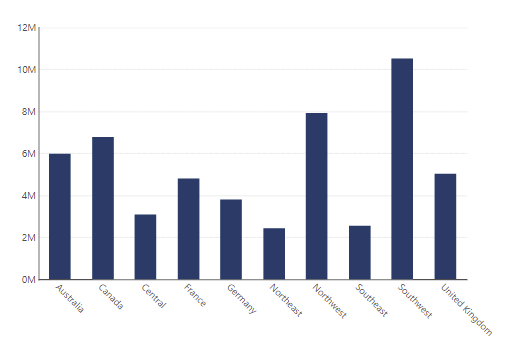Formula - Percent of Total input data

## 3. Parameters

The Percent of Total function requires the following parameters:

• Alignments... (One or More, Optional) – Hierarchy placeholders to be used as the alignment axis.

## 4. Output

The Percent of Total function generates a result set in a decimal format, where each value represents a portion of the total (1).

### 4.1. Change the presentation (optional)

For example, a common practice is to use the calculated values for percentage labels:

1. Change the formatting on the measure.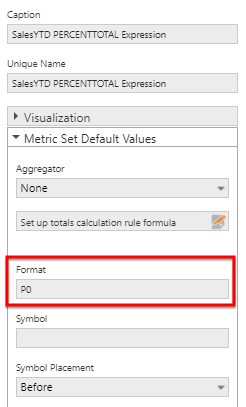Format the measure
2. Hide the measure.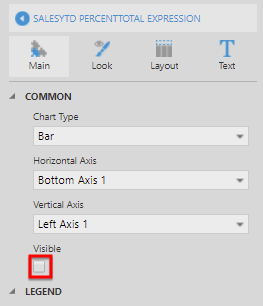Hide the measure
3. Add the measure values to the data point labels.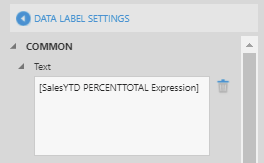Add to the data point labels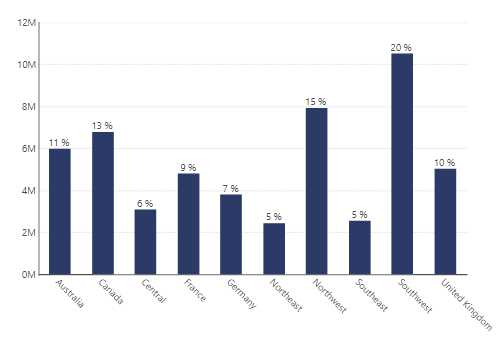Show the Percent of Total as labels